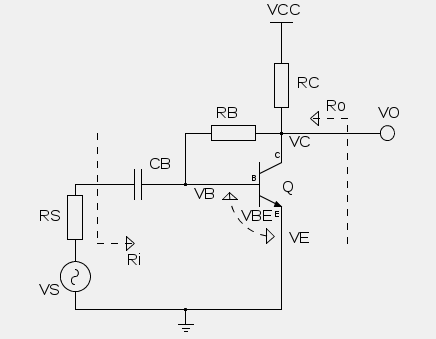OVERVIEW This is a simple design tool for calculating bias resistor values, small-signal gain and input/output resistances of a common-emitter BJT amplifier with collector-to-base feedback. Just fill the input fields below in given order from top to bottom. The ordering of the fields serves as a step-by-step guide for the design process. The base bias resistor values are calculated automatically (using 5% tolerance resistances), but custom values can be given and those will not be overwritten. The 'Calculate values' button in the bias resistor field always rounds up to the nearest 5% tolerance, even user defined values. The small-signal values are evaluated in the mid-band, CB short-circuited. The small-signal gain is evaluated as VO/VS, and the input/output resistances are evaluated as shown in the pic.Select the operating voltage VCC. Typically 9 volts is the way to go. VCC : volts RESULTS DC Bias voltages: VC : volts VE : volts VB : volts AC Gain factor: Av : const Input resistance: Ri : ohms Output resistance: Ro : ohms Select the BJT you want to use. From the datasheet of the chosen transistor, lookup for the typical current gain factor and place it here as a parameter. hFE : const For convenience, you can change the base-emitter voltage, which currently is set to 0.65 V by default. Typically VBE is around 0.55 - 0.75 V. VBE : volts Choose the collector bias voltage to aim with the biasing design. In a normal design the collector bias voltage is half of the operating voltage VCC. VC : volts Next select a value of the collector resistor. RC should be mainly chosen to limit collector current to the suitable level. The value of RC affects the base bias resistor values and output impedance. RC : ohms The biasing is finalized by setting a feedback resistor between collector and base. By pressing the button below, the bias resistor value is calculated automatically. RB : ohms Also, the internal resistance of the signal source should be given. For ideal source this value is small, but for guitar output this is a few kilohms. RS : ohms